# NAME

Math::Window2Viewport - Just another window to viewport mapper.

# SYNOPSIS

``````  use Math::Window2Viewport;

my \$mapper = Math::Window2Viewport->new(
Wb => 0, Wt => 1, Wl => 0, Wr => 1,
Vb => 9, Vt => 0, Vl => 0, Vr => 9,
);

my (\$x, \$y) = (0.5, 0.6);
my \$x2 = int( \$mapper->Dx( \$x ) );
my \$y2 = int( \$mapper->Dy( \$y ) );``````

# DESCRIPTION

This module will convert one set of coordinates (the World Window) into another set (the Viewport) for the purposes of graphing any set of points from one system to another.

# METHODS

• `new()`

Constructs object. Required parameters:

``````         Wt                  Vt
+----------+       +------------+
|          |       |            |
Wl|  window  |Wr   Vl|  viewport  |Vr
|          |       |            |
+----------+       +------------+
Wb                  Vb``````
• `Wb`

``  world window bottom``
• `Wt`

``  world window top``
• `Wl`

``  world window left``
• `Wr`

``  world window right``
• `Vb`

``  viewport bottom``
• `Vt`

``  viewport top``
• `Vl`

``  viewport left``
• `Vr`

``  viewport right``
• `Dx( x )`

Calculates new point `Dx` for given point `x`. Client is responsible for casting value to int.

• `Dy( y )`

Calculates new point `Dy` for given point `y`. Client is responsible for casting value to int.

# EXAMPLE

The following will generate a Fourier synthesized square wave via GD::Simple:

``````  use GD::Simple;
use Math::Window2Viewport;

my (\$width, \$height, \$res) = (500, 300, .02);
my \$img = GD::Simple->new( \$width, \$height );
my \$mapper = Math::Window2Viewport->new(
Wb => -1, Wt => 1, Wl => -1, Wr => 1,
Vb => \$height, Vt => 0, Vl => 0, Vr => \$width,
);

my (%curr,%prev);
for (my \$x = -1; \$x <= 1; \$x += \$res) {
my \$y = 0;
for (my \$i = 1; \$i < 20; \$i += 2) {
\$y += 1 / \$i * cos( 2 * 3.1459 * \$i * \$x + ( -3.1459 / 2 ) );
}
%curr = ( dx => \$mapper->Dx( \$x ), dy => \$mapper->Dy( \$y ) );
\$img->moveTo( @prev{qw(dx dy)} );
\$img->lineTo( @curr{qw(dx dy)} );
%prev = %curr;
}

print \$img->png;``````

Try changing the value for `\$res`. See the example directory for more.

# RENDERED EXAMPLES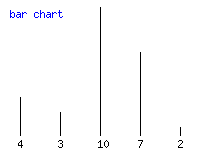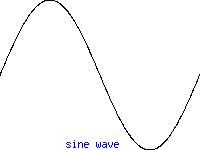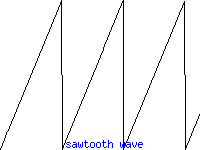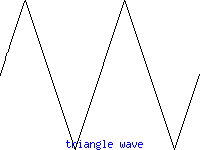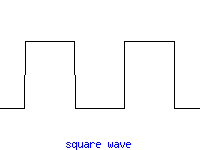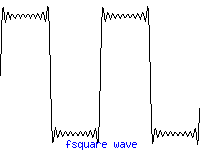# AUTHOR

Jeff Anderson, `<jeffa at cpan.org>`

This program is free software; you can redistribute it and/or modify it under the terms of the the Artistic License (2.0). You may obtain a copy of the full license at: